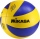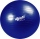# What is bigger?

Which ball has a larger volume: a football with a circumference of 66 cm or a volleyball with a diameter of 20 cm?

Result

x = (Correct answer is: F)### Step-by-step explanation:Did you find an error or inaccuracy? Feel free to write us. Thank you!Blossom
I love this website so much. And i am doing math  work from Home. Because of the Covid-19Blossom
I need help now.Tips to related online calculators
Tip: Our volume units converter will help you with the conversion of volume units.

#### You need to know the following knowledge to solve this word math problem:

We encourage you to watch this tutorial video on this math problem:

## Related math problems and questions:

• The volleyball ballThe volleyball ball can have a circumference after inflation of at least 650 max 750 mm. What volume of air can this ball hold, if its circumference is the average of the minimum and maximum inflation of the ball.
• Sphere floatingWill float a hollow iron ball with an outer diameter d1 = 20cm and an inside diameter d2 = 19cm in the water? The iron density is 7.8 g/cm3. (Instructions: Calculate the average sphere density and compare it with the water density. )
• The inflatedThe inflated gymnastic ball should have a diameter of 65 cm. How many times do we have to pump air into a fully blown ball with a bicycle inflator to inflate it if the working volume of the inflator is a cylinder with an inner diameter of 2 cm and a heigh
• EightEight small Christmas balls with a radius of 1 cm have the same volume as one large Christmas ball. What has a bigger surface: eight small balls, or one big ball?Calculate the radius of the circle whose length is 107 cm larger than its diameter
• Arm-legCalculate the length of the base of an isosceles triangle with a circumference 224 cm if the arm length is 68 cm.
• The ballThe ball has a radius of 2m. What percentage of the surface and volume is another sphere whose radius is 20% larger?
• The potThe pot is a cylinder with a volume of V = 7l and an inner diameter of d = 20cm. Find its depth.
• Perimeter of baseThe circumference of the base of the rotating cylinder is same as its height. What is the diameter and height of this cylinder with volume 1 liter?
• MO SK/CZ Z9–I–3John had the ball that rolled into the pool, and it swam in the water. Its highest point was 2 cm above the surface. The diameter of the circle that marked the water level on the surface of the ball was 8 cm. Find the diameter of John ball.
• CircleHow big is an area of a circle if its circumference is 51.2 cm?
• Circle - simpleThe circumference of a circle is 198 mm. How long in mm is its diameter?
• Second sideCalculate the length of the other side of the rectangle if its circumference is 60 cm and one side is 10 cm long.
• Steel tubeThe steel tube has an inner diameter of 4 cm and an outer diameter of 4.8 cm. The density of the steel is 7800 kg/m3. Calculate its length if it weighs 15 kg.
• The prison ballCalculate the density of the material that the prison ball is made from if you know its diameter is 15cm and its weight is approximately 2.3kg. With the help of mathematical-physicochemical tables estimate what material the ball is made from.
• Rectangle 45The perimeter of a rectangle is 60cm. If the length of the rectangle is 20cm. a)find the width b)find the area.
• Sphere parts, segmentA sphere with a diameter of 20.6 cm, the cut is a circle with a diameter of 16.2 cm. .What are the volume of the segment and the surface of the segment?